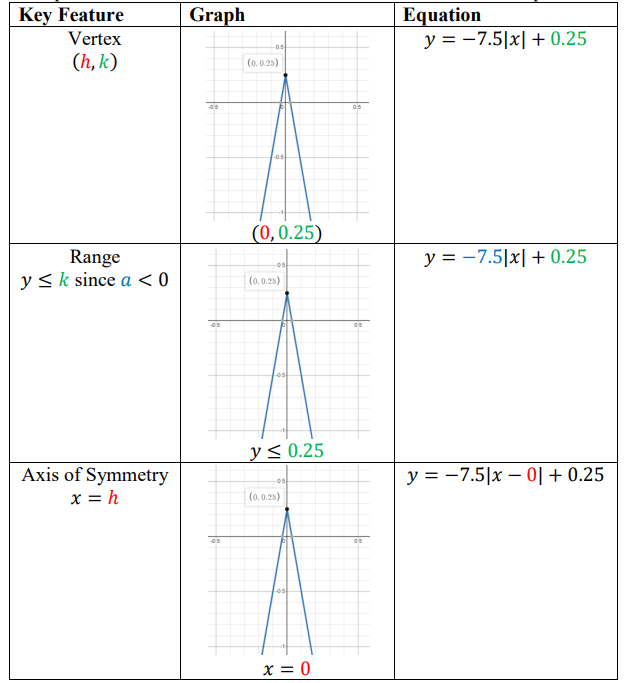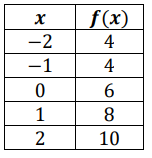# MA.912.AR.4.3Export Print
Given a table, equation or written description of an absolute value function, graph that function and determine its key features.

### Clarifications

Clarification 1: Key features are limited to domain; range; intercepts; intervals where the function is increasing, decreasing, positive or negative; vertex; end behavior and symmetry.

Clarification 2: Instruction includes representing the domain and range with inequality notation, interval notation or set-builder notation.

Clarification 3: Within the Algebra 1 course, notations for domain and range are limited to inequality and set-builder.

General Information
Subject Area: Mathematics (B.E.S.T.)
Strand: Algebraic Reasoning
Status: State Board Approved

## Benchmark Instructional Guide

### Terms from the K-12 Glossary

• Absolute Value
• Coordinate Plane
• Domain
• Function Notation
• Piecewise Function
• Range
• $x$-intercept
• $y$-intercept

### Vertical Alignment

Previous Benchmarks

Next Benchmarks

### Purpose and Instructional Strategies

In middle grades, students graphed linear equations in two variables. In Algebra I, students graph absolute value functions and determine key features. In later courses, students will solve real-world problems involving absolute value functions and piecewise functions.
• In Algebra I, for mastery of this benchmark use $y$ = $a$|$x$$h$| + $k$ where  $a$ is nonzero and $h$ and $k$ are any real number.
• The vertex of the graph is ($h$, $k$).
• The domain of the graph is set of all real numbers and the range is $y$$k$ when $a$ > 0.
• The domain of the graph is set of all real numbers and the range is $y$$k$ when $a$ < 0.
• The axis of symmetry is $x$ = $h$
• The graph opens up if $a$ > 0 and opens down if $a$ < 0.
• The graph $y$ = |$x$| can be translated $h$ units horizontally and $k$ units vertically to get the graph of $y$ = $a$|$x$$h$| + $k$
• The graph $y$ = $a$|$x$| is wider than the graph of $y$ = |$x$| if |$a$| < 1 and narrower if |$a$| > 1.
• Instruction includes the understanding that a table of values must state whether the function is an absolute value function.
• For example, if given the function $y$ = |$x$| and only positive values of $x$ were given in a table, one would only have part of the graph. Discuss the importance of providing enough points in a table to create an accurate graph.
•  When making connections to transformations of functions, use graphing software to explore $y$ = $a$|$x$$h$| + $k$ adding variability to the parent equation to see the effects on the graph. Allow students to make predictions (MTR.4.1).
•  Instruction provides opportunities to make connections to linear functions and its key features.
•  Instruction includes the use of $x$-$y$ notation and function notation.
•  Instruction includes representing domain and range using words, inequality notation and set-builder notation.
• Words If the domain is all real numbers, it can be written as “all real numbers” or “any value of $x$, such that $x$ is a real number.”
• Inequality notation If the domain is all values of $x$ greater than 2, it can be represented as $x$ > 2.
• Set-builder notation If the domain is all values of $x$ less than or equal to zero, it can be represented as {$x$|$x$ ≤ 0} and is read as “all values of $x$ such that $x$ is less than or equal to zero.”
• When addressing real-world contexts, the absolute value is used to define the difference or change from one point to another. Connect the graph of the function to the real-world context so the graph can serve as a model to represent the solution (MTR.6.1, MTR.7.1).
• Instruction includes the use of appropriately scaled coordinate planes, including the use of breaks in the $x$- or $y$-axis when necessary.

### Common Misconceptions or Errors

• Students may not fully understand the connection of all of the key features (emphasize the use of technology to help with student discovery) and how to represent them using the proper notation.

### Strategies to Support Tiered Instruction

• Teacher models using a graphing tool or graphing software to help students discover the key features and their connections to the absolute value equation.
• Teacher provides a colored visual of a two-variable absolute value equation and its graph.• Graph the function $f$($x$) = −$\frac{\text{1}}{\text{2}}$|$x$ − 4| + 6 and determine its domain; range; intercepts; 2 intervals where the function is increasing, decreasing, positive or negative; vertex; end behavior and symmetry.

### Instructional Items

Instructional Item 1
• Given the table of values for an absolute value function, graph the function.*The strategies, tasks and items included in the B1G-M are examples and should not be considered comprehensive.

## Related Courses

This benchmark is part of these courses.
1200310: Algebra 1 (Specifically in versions: 2014 - 2015, 2015 - 2022, 2022 and beyond (current))
1200320: Algebra 1 Honors (Specifically in versions: 2014 - 2015, 2015 - 2022, 2022 and beyond (current))
1200370: Algebra 1-A (Specifically in versions: 2014 - 2015, 2015 - 2022, 2022 and beyond (current))
1200400: Foundational Skills in Mathematics 9-12 (Specifically in versions: 2014 - 2015, 2015 - 2022, 2022 and beyond (current))
7912080: Access Algebra 1A (Specifically in versions: 2014 - 2015, 2015 - 2018, 2018 - 2019, 2019 - 2022, 2022 and beyond (current))
1200315: Algebra 1 for Credit Recovery (Specifically in versions: 2014 - 2015, 2015 - 2022, 2022 and beyond (current))
1200375: Algebra 1-A for Credit Recovery (Specifically in versions: 2014 - 2015, 2015 - 2022, 2022 and beyond (current))
7912075: Access Algebra 1 (Specifically in versions: 2014 - 2015, 2015 - 2018, 2018 - 2019, 2019 - 2022, 2022 and beyond (current))

## Related Access Points

Alternate version of this benchmark for students with significant cognitive disabilities.
MA.912.AR.4.AP.3: Given a table, equation or written description of an absolute value function, select the graph that represents the function.

## Related Resources

Vetted resources educators can use to teach the concepts and skills in this benchmark.

## Student Resources

Vetted resources students can use to learn the concepts and skills in this benchmark.

## Parent Resources

Vetted resources caregivers can use to help students learn the concepts and skills in this benchmark.